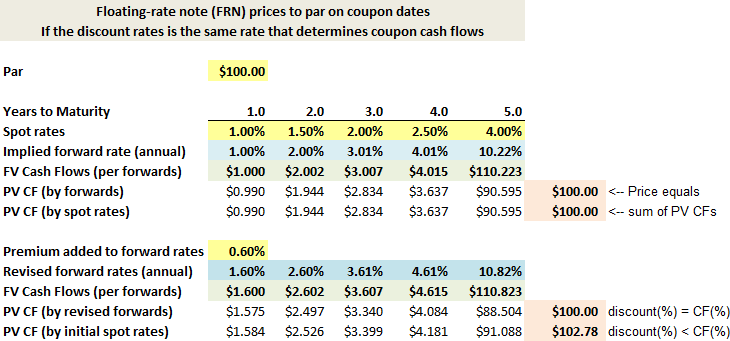# YouTubeT3-32: Valuation of plain-vanilla interest rate swap

#### Nicole Seaman

##### Director of FRM Operations
Staff member
Subscriber
David breaks down the valuation of an interest rate swap into three steps: 1. The assumptions, which includes understanding the TIMELINE; e.g., we are valuing the stop at some point after origination and it has some remaining life (in this case 15 months); 2. Extracting the implied semi-annual forward rates from the LIBOR zero rate curve; and 3. Modeling the cash flows, in the first part as a series of forward rate agreements (FRAs).

David's XLS is here: https://trtl.bz/2Q4XFCh

Last edited:

#### David Harper CFA FRM

##### David Harper CFA FRM
Staff member
Subscriber
FAQ: Why does a floater price to par on coupon settlement dates; aka, how are we able to value the floating-rate leg of the swap as a single cash flow when it's really a stream of future cash flows?

About the valuation of an interest rate swap "as if two bonds," a very popular question we get is: why is it that we can value the floating-rate leg by assuming only one cash flow? At first, it does seem strange given that the floating-rate leg of a swap will pay several coupons in the future (not just one)! The assumption, as I start to review in the video above (at about 15:00) is that a floating-rate note (FRN) prices exactly to par at each coupon settlement date. Put another way, you should be willing to pay $100.00 (or$1,000.00; i.e., face value) for a note/bond with a coupon that floats, if the floating rate used to determine the coupon (e.g., LIBOR) is the same rate that you will use as your discount rate (ie., to discount your expected future cash flows).

This is not immediately intuitive to many of us. Although for some people it probably is intuitive: first imagine you buy a 10-year fixed-rate annual-pay bond with a 4.0% per annum coupon rate while the (spot rate) term structure is conveniently flat at 4.0%; you will pay exactly $100.00; i.e., if coupon rate equals yield, the bond's price is par because the interest (the coupons) covers the yield, no more and no less, so you don't require any expected capital appreciation. If the interest rate increases to 5.0%, the price of your bond drops (per the inverse relationship between price and yield) because, if you want to sell the bond in the "new" 5.0% rate environment, nobody will pay$100.00 for it. Why would anybody pay "full price" for a bond yielding 4.0% if the new yield is 5.0%?! No, they will only pay, and you can only sell, at the price given by -PV(yield = 5%, 10, coupon = 4%, $100) =$92.28. The buyer now expects a 5.0% yield and they're getting $4.00/92.28 = 4.33% from the coupon plus the other ~ 0.67% from the expected capital appreciation as the bond will pull-to-par and redeem at$100.00 (it's actually not the only way to parse these components but that's not the topic here). In any case, in the case of a typical vanilla bond, the price goes down when the interest rate goes up because a lower price is required to make the same coupon stream competitive at the new interest rate. But if the coupons floats up or down in tandem with the discount rate, then the bond price does not need to adjust! If your bond is instead a floater and the interest rate increases from 4.0% to 5.0%, then the coupon increases also: the new coupon "handles" the entire yield need, no price adjustment is necessary. The price only needs to adjust during the time between coupons, when the coupon rate and the discount rate temporarily diverge.

Below I tried to illustrate why a floater must price to par at each coupon date. Very simple illustration. The XLS is here at https://www.dropbox.com/s/hwrv6m6svt5eqwu/070420-floater-prices-to-par.xlsx?dl=0

The only inputs are in yellow. The upper panel imagines a spot rate term structure {1.00% at 1.0 year, 1.50% at 2.0 years, ..., 4.00% at 5.0 years}. The spot rate term structure informs the forward rate term structure (in blue). The expected future cash flows are based on the forward rates; i.e., the forward rates, if unbiased, represent the expected future one-year spot rates. If we discount those cash flow, the bond's price is exactly \$100.00. Put simply, if the coupon cash flows are paid at the same rate that we use to discount the cash flows, the bond prices to par. That's the idea.

In the lower panel, I simply added a 60 basis premium to the forward rate term structure. Then DCF valued the bond. First, again, if discounted at the revised forward rates, the price is (again) par. But second (bottom row), I discounted the revised cash flows at the original spot rates (which is also the same as discounting at the original forward rate); in this case, the bond becomes a premium-priced bond. That's because the discount rates are lower than the rates used to determine the cash flow. I hope that's interesting.Last edited: